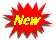HTML3 Problems on Number System For SSC CGL, CHSL, BANKS Repeatedquestions

### Problems on Number System For SSC CGL, CHSL, BANKS

Q. Product of two consecutive odd numbers is 6723. Find the square root of smallest number ?

a. 49
b. 43
c. 9
d. 11

---------------------------------------------------------------------------
Q. Product of two consecutive Even Numbers is 6888. Find the sqaure of largest number?

a. 5456
b. 7056
c. 3245
d. 2025

---------------------------------------------------------------------------
Q. Product of two consecutive number is 5256 . Find sum of both number ?

a. 145
b. 79
c. 135
d. 195

---------------------------------------------------------------------------
Q. What is 309 times 323?

a. 9804
b. 99807
c. 96542
d. 67584

---------------------------------------------------------------------------

Q.Sum of square of two consective even numbers is 1060. Find smallest number?

a. 22
b. 24
c. 26
d. 18

---------------------------------------------------------------------------

Q.Sum of digits of two digit number is 15 and difference of digits is 3. Find Product of digits?

a. 51
b. 58
c. 63
d. 54

---------------------------------------------------------------------------

Q. Difference of two two-digit number is 18. Four times the second number is 18 more than thrice the first number. Find the sum of both numbers?

a. 162
b. 165
c. 89
d. 48

---------------------------------------------------------------------------

Q. A number when five times multiply by itself becomes 3125. Find number??

a. 5
b. 6
c. 7
d. 15

---------------------------------------------------------------------------

Q. When 3626 divided by square of a number and remainder multiplied by 32 answer so obtained is 2368. Find number??

a. 5
b. 14
c. 7
d. 13

---------------------------------------------------------------------------

Q. Product of two Consecutive prime numbers is 3127. Find largest number ?

a. 59
b. 57
c. 53
d. 55

---------------------------------------------------------------------------

Q. Five bells begin to toll together at intervals of 9seconds, 6seconds, 4seconds, 10seconds and *seconds. How many times will they toll together in the span of 1hour(Excluding the toll at the start) ?

a. 5
b. 8
c. 10
d. NoneWe Need Your Valuble Feedback in comments